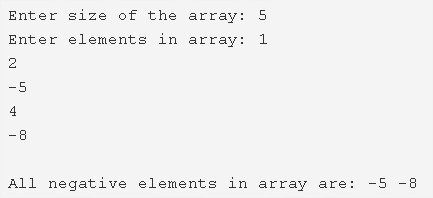# C Program To Print All Negative Elements In An Array

C Program to print all negative elements in an array – In this article, we will detail in on how to print all the negative elements present in an array in C programming language.

Suitable examples and sample programs have also been added so that you can understand the whole thing very clearly. The compiler has also been added with which you can execute it yourself.

The various means used in this piece are as follows:

• Using Standard Method
• Using Function
• Using Recursion

As you all know, an array is an arrangement or a sequence of a group of elements, be it digits or alphabets or anything else. In this case, we have to find a way to print all the negative elements present in an array.As you can see, in the above example, 5 elements are present in the given array. Those are:

• 1
• 2
• -5
• 4
• -8

Out of these, two are negative in nature, i.e. -5 and -8. Those two elements will get printed only.

Thus, the methods to print all the negative elements in an array in C programming are as follows:

## Using Standard Method

1. Read the entered ‘ array size’ and store that value into the variable n.

2) Read the entered array elements using scanf() function, and store the array elements into the array a[] using for loop with the structure for(i=0;i<n;i++).

3) Compare each element of an array with zero as a[i]<0 ,using for loop with the structure for(i=0; i<n;i++).If any element of an array is less than zero then print that element which is negative.

4) After all iterations of for loop, we will get all negative elements of an array.

Output:

### Using Function – C Program To Print All Negative Elements In An Array

1. Any number which is less than zero is a negative number. Here, we are using a function printnegativenumbers() to find the negative elements of the given array.

2) Pass an array, size of the array to the function printnegativenumbers() as parameters.

4) printnegativenumbers() function will compare each array element with 0 as a[i]<0 using for(i=0;i<n;i++) loop. If any element is less than zero then it prints that element which is negative.

Output:

#### Using Recursion – C Program To Print All Negative Elements In An Array

1. A function which calls itself is a recursive function until some condition becomes false.

2) The function printnegativenumbers() calls itself as printnegativenumbers(a,n,++i),so printnegativenumbers() is the recursive function.

3) The main() function calls the printnegativenumbers() function by passing array,size of the array,i value as printnegativenumbers(a,n,0).

4) Then the function checks the condition i<n.

If the condition i<n is true

then it compares each element of the array with zero and prints the negative number which is less than zero and calls the function itself by increasing i value, until i<n condition becomes false.

Output:

x

## C Program To Print Number Of Days In A Month | Java Tutoring

C program to input the month number and print the number of days in that ...